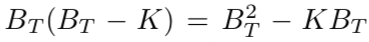top of page
Search

# The Original BitMEX Bitcoin Quanto Forward Contract

Updated: Jun 14, 2021

The BitMEX cryptocurrency derivatives exchange launched sometime around late 2014.

At the time, a small number of exchanges had implemented Bitcoin forward contracts that offered linear exposure to the Bitcoin price. The way they actually tended to define such contracts was to define the contract size in terms of USD, and implement a forward purchase of Bitcoin for USD as a forward sale of USD denominated in Bitcoin.

The original forward BTC/USD contract BitMEX listed at launch was defined quite differently. A long position in the contract settled as follows: if S is the settlement Bitcoin price and K is the forward price paid, then the numeric quantity S - K is then multiplied by some constant factor defined in the contract specs. The long contract owner is then credited or debited that number of bitcoins.

Therefore, the contract was a quanto: it took the value of a USD-denominated asset or contract (such as a standard linear forward contract) and simply converts the value at a fixed exchange rate to some other currency (Bitcoin in this case).

The settlement price was defined as the arithmetic average price, sampled every minute over the 2 hours prior to settlement. Given a substantial amount of time remaining prior to settlement, the spot price dynamics prior to the averaging window dominate the dynamics or distribution of the settlement price, so in modeling this contract we will as an approximation assume it simply settles against the spot price.

We also assume here Bitcoin pays no dividends. (That was true for a long time, and in particular when BitMEX launched, but now not so much.) For convenience and notation reduction we assume 0 interest rates as well - generalizing to non-0 rates is relatively straightforward.

The effect of the quanto specification is to essentially manufacture a forward contract in which the quantity is proportional to the Bitcoin USD price B. Without loss of generality we assume the proportionality constant is 1. The USD value at settlement isAs is typical for such modeling, we assume no arbitrage and there exists an equivalent martingale measure for the spot price. Then the current value is the expected settlement value under a/the martingale measure:The first term shows there is clearly positive convexity in this contract. Basically it's because both the effective contract quantity and P&L increase with the Bitcoin price.

The fair forward price corresponds to setting the above to 0:The fair forward price can also be written asObservations:

1. The fair value is always greater than the current spot price.

2. The fair value increases with time to settlement.

## Black Scholes Valuation

Under Black Scholes assumptions the martingale measure is unique and the spot price follows Geometric Brownian Motion. In this case the above equations can be solved exactly given the volatility:where tau is the time until settlement. Definingthe value, fair value and various greeks are thenObservations:

1. If the spot price is sufficiently less than the forward purchase price, a long contract holder is actually short the spot.

2. For a given fixed volatility, the gamma is independent of the spot.

3. Both the vega and gamma increase with time to settlement. A long calendar spread will be both long vega and gamma.

As can be done for vanilla calls and puts, a Black Scholes volatility can be implied from the observed market price. For this contract the implied volatility isObservations:

1. For a given volatility, the term structure of fair values increases exponentially as a function of the term and in particular is convex. This is much different than they type of contango you typically see in other forward markets.

2. Because vol convexity is positive everywhere, the implied volatility should be greater than the expected asset volatility over the term, and greater than the implied volatility of a vanilla option with the same term.

At the time BitMEX launched and listed this contract, the platform was essentially exclusively populated with retail customers. Though the above modeling would be straight-forward for e.g. a bank derivatives desk, it would've been largely unknown to the exchange's customers at the time. As a consequence, the implied volatility was highly random even over short time periods. For example the mid implied vol could be, say, 40% at one time and then several hours later around 100%, or vice versa, with no apparent fundamental or economic explanation for such movement.